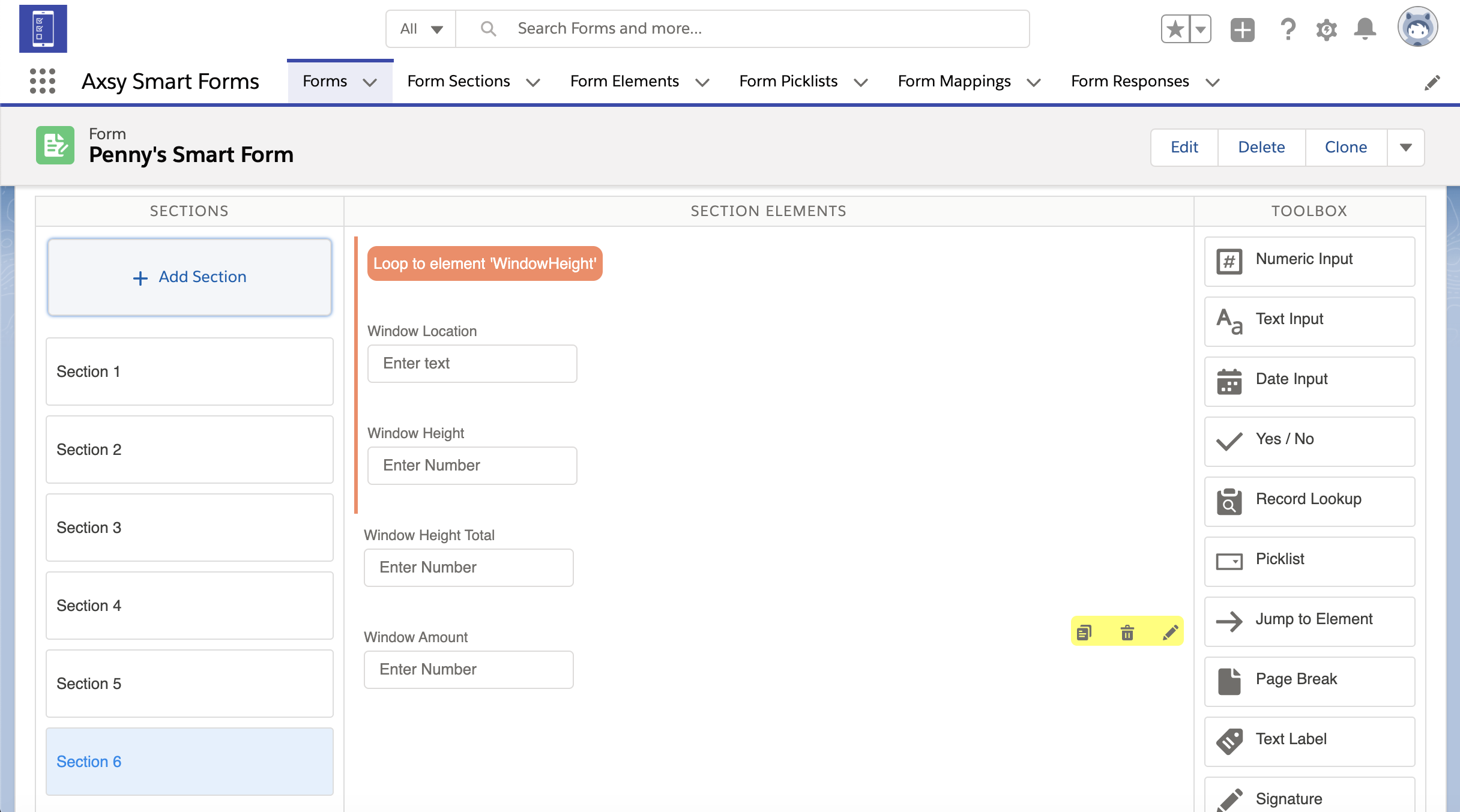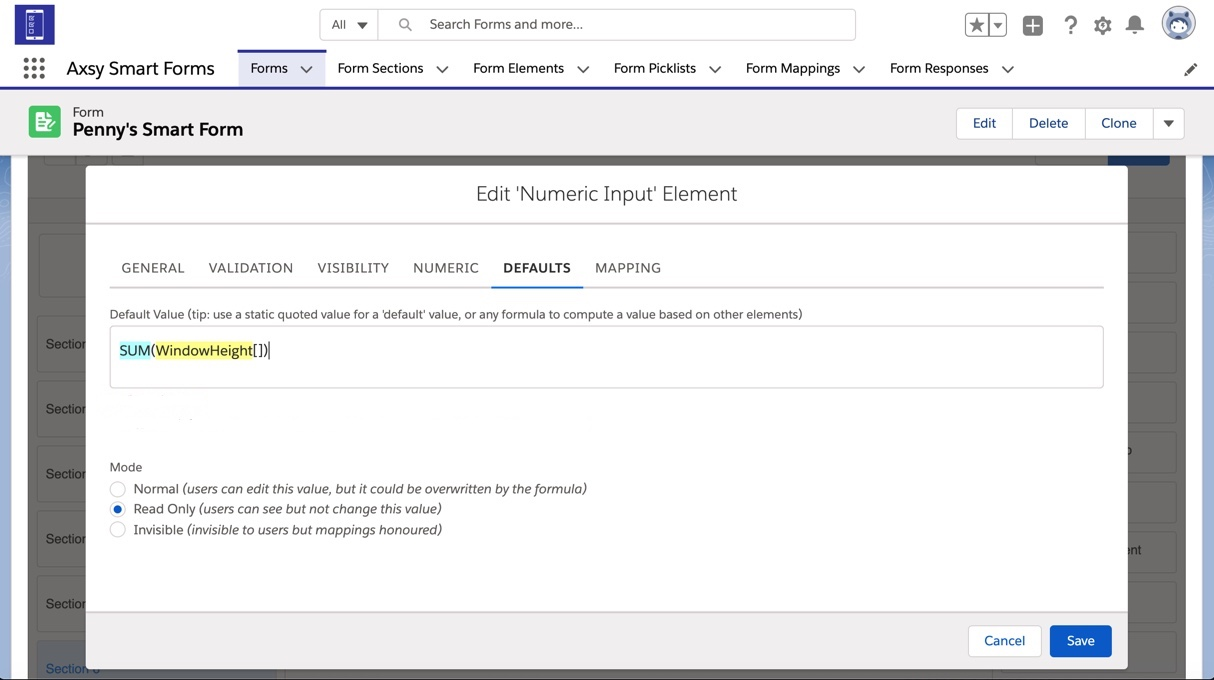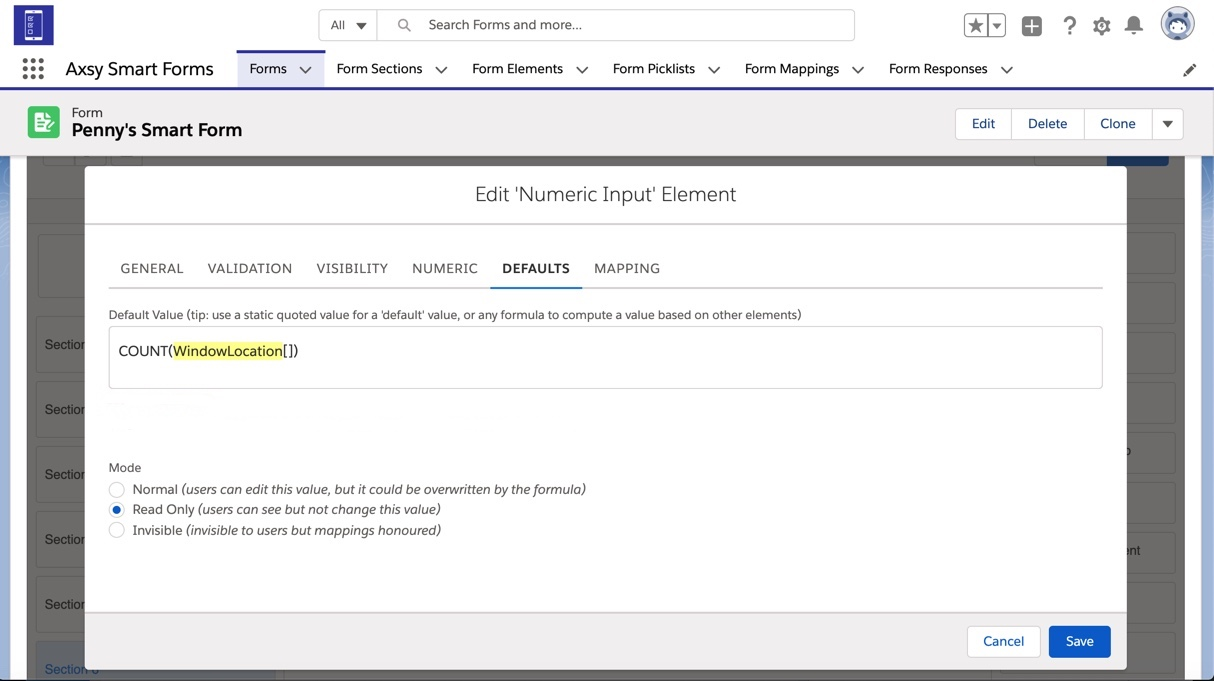It is possible to calculate SUM and COUNT totals for the accumulation of a specific Element in a Loop. For more information on Loop Elements, please see this article: Configuring Loops

Elements that come after a Loop Element can make use of these roll-up calculations in their formulas by using the following syntax: SUM(ElementName[]) and COUNT(ElementName[]), where ElementName refers to an element encapsulated by a Loop Element that needs to be summed or counted - Don't forget the square brackets [] !

It should also be noted that these formulas only work with specific Element Types:

1. SUM works only with Numeric Elements
2. COUNT works with Text, Numeric, Picklist and Date Elements

Example Roll-Up Formulas

Here is a Loop about windows. This example Form includes:

1. Two Elements within the Loop that contain the window height and location: 'WindowHeight' and 'WindowLocation'.
2. Two Elements outside of the Loop for calculating the total number of windows and total height of all of the windows: 'TotalWindowCount' and 'TotalWindowHeight'.1. To find the total SUM of the height of the windows, you can use a formula for the Default Value of the TotalWindowHeight element and reference the 'WindowHeight' element field encapsulated by the Loop Element. Use the Formula: 'SUM(WindowHeight[])' to add up all the values entered in the WindowHeight element field for all loop iterations and populate a total.2. You can find the total COUNT of the amount of windows data has been recorded for by referencing any of the elements within the Loop. Use the formula: 'COUNT(WindowLocation[])' to count the number of times 'WindowLocation' has been displayed in the Loop and populate how many windows there are in total. .